# RD Sharma Solutions For Class 12 Maths Exercise 4.7 Chapter 4 Inverse Trigonometric Functions

RD Sharma Solutions Class 12 Maths Exercise 4.7 Chapter 4 Inverse Trigonometric functions is provided here. Students can use the PDF of solutions to get their doubts cleared instantly and solve problems as per the latest exam pattern. It helps students to self analyse their knowledge about the concepts covered under each exercise of Chapter 4.

To perform better in the board exam, students can make use of RD Sharma Solutions Class 12 Maths Chapter 4 Inverse Trigonometric functions Exercise 4.7, from the links which are given here. This exercise deals with the properties of Inverse Trigonometric Functions.

## Download the PDF of RD Sharma Solutions For Class 12 Chapter 4 – Inverse Trigonometric Functions Exercise 4.7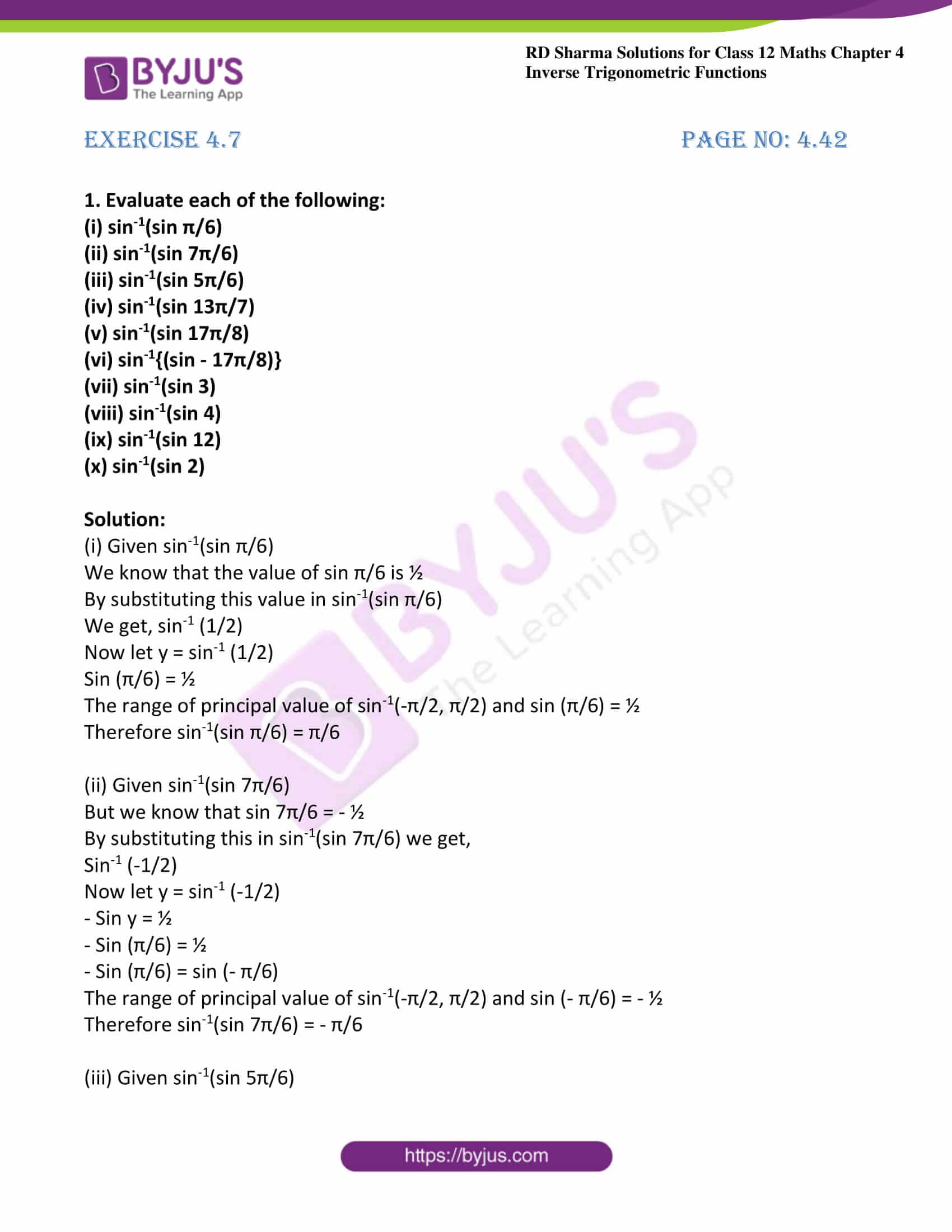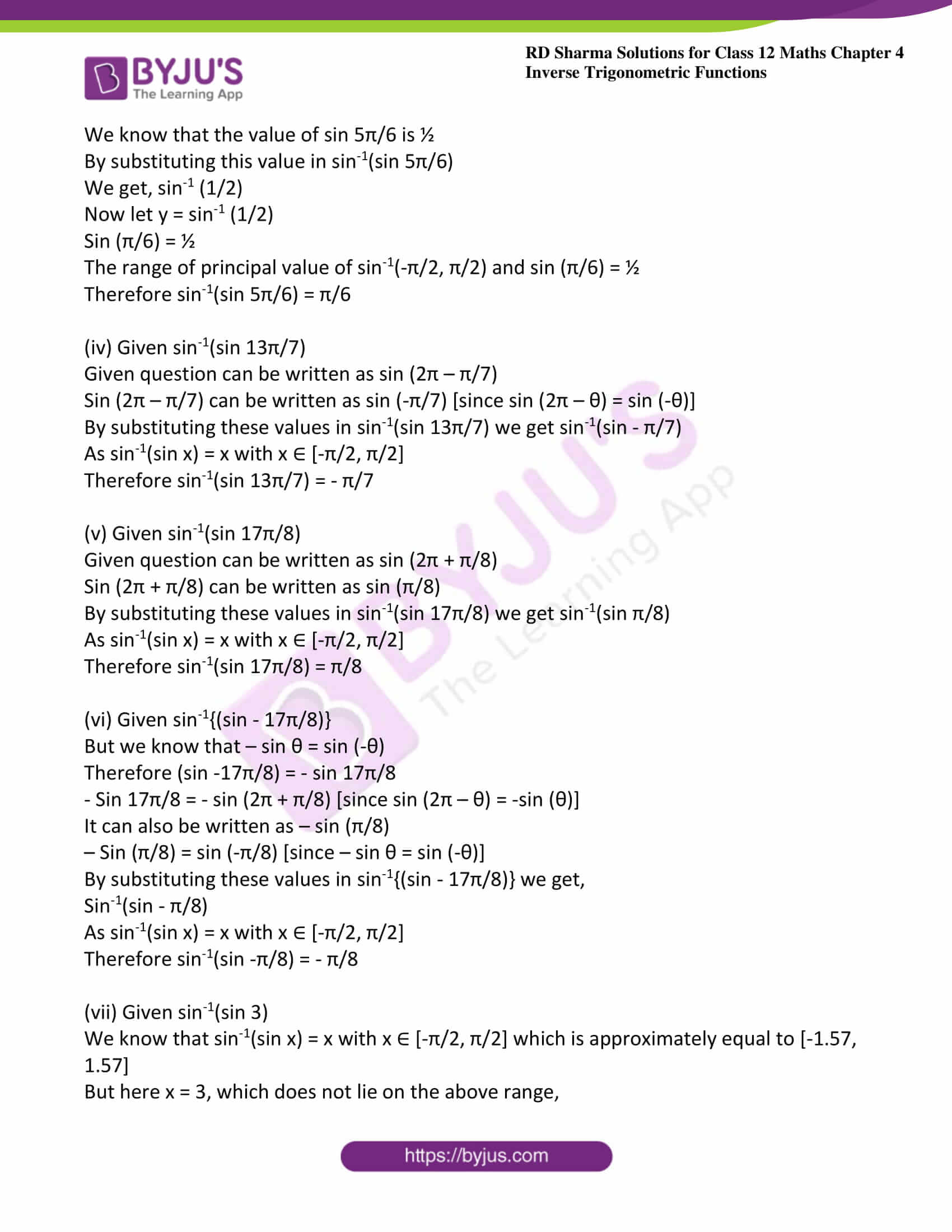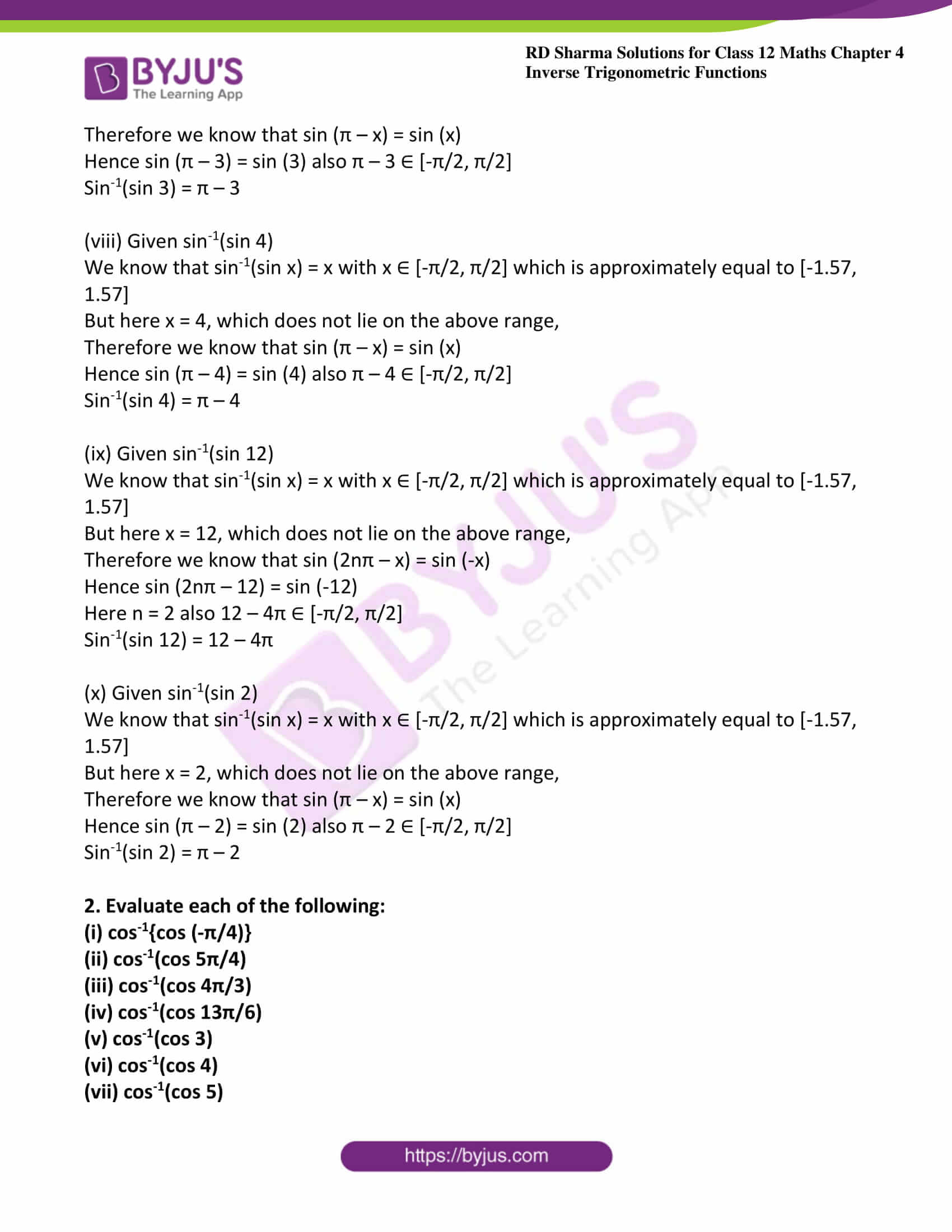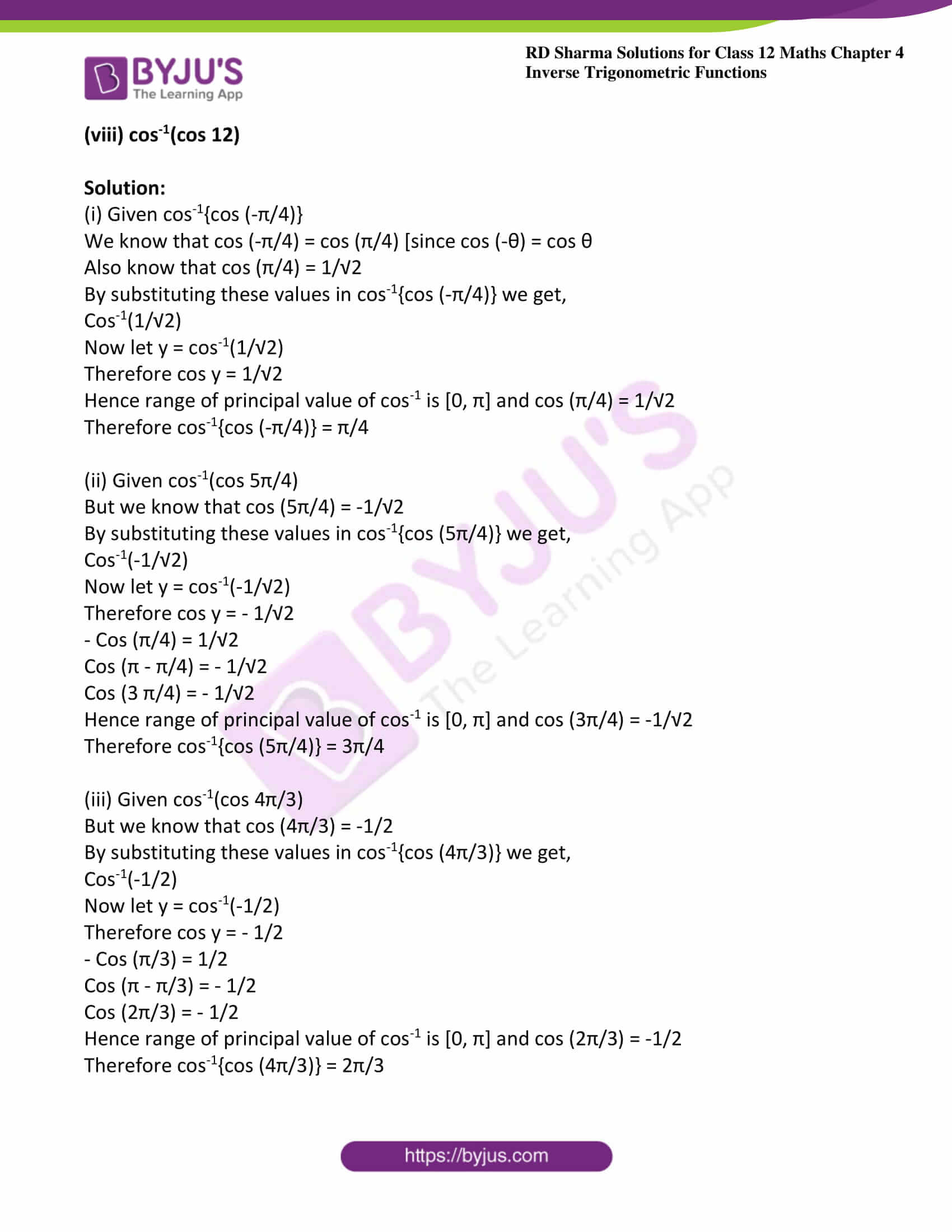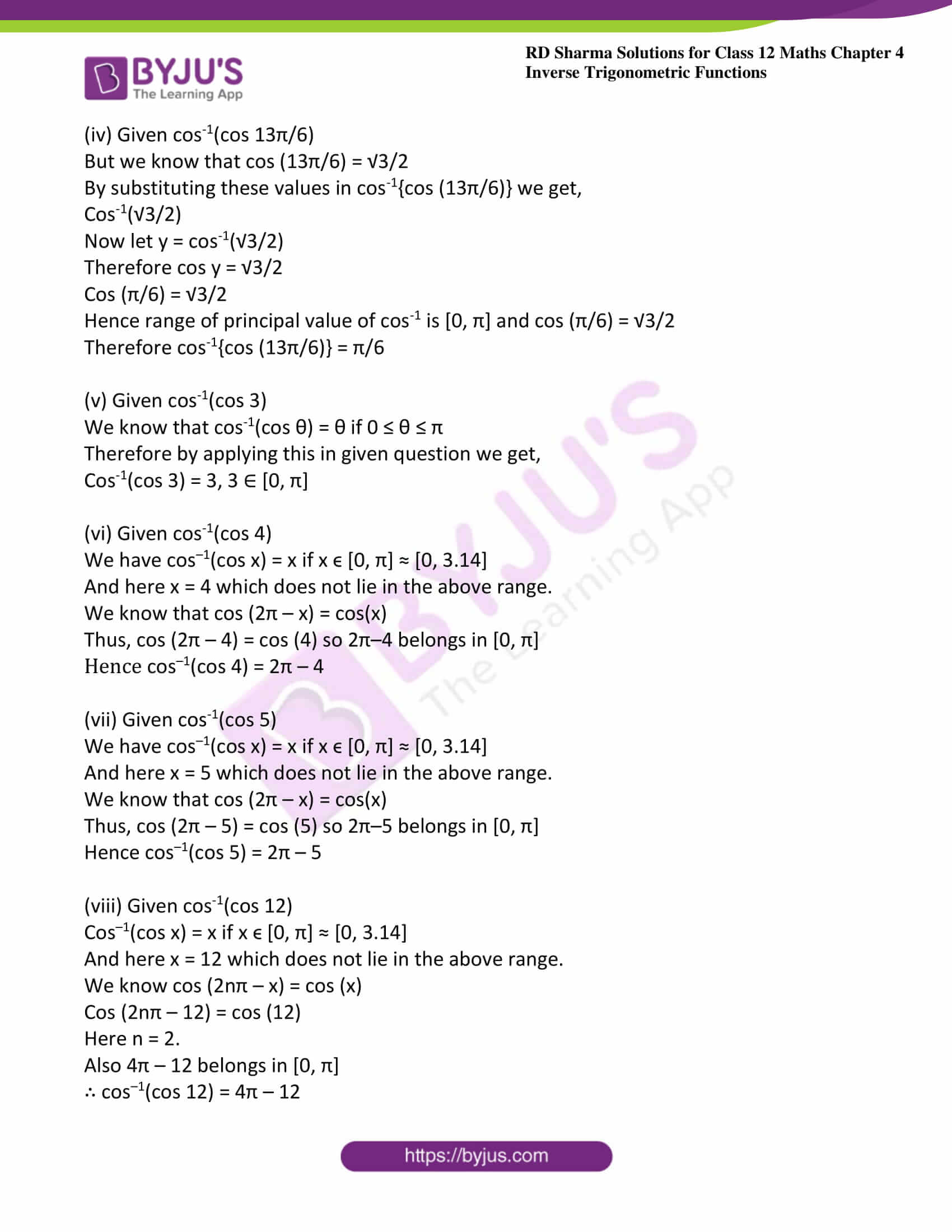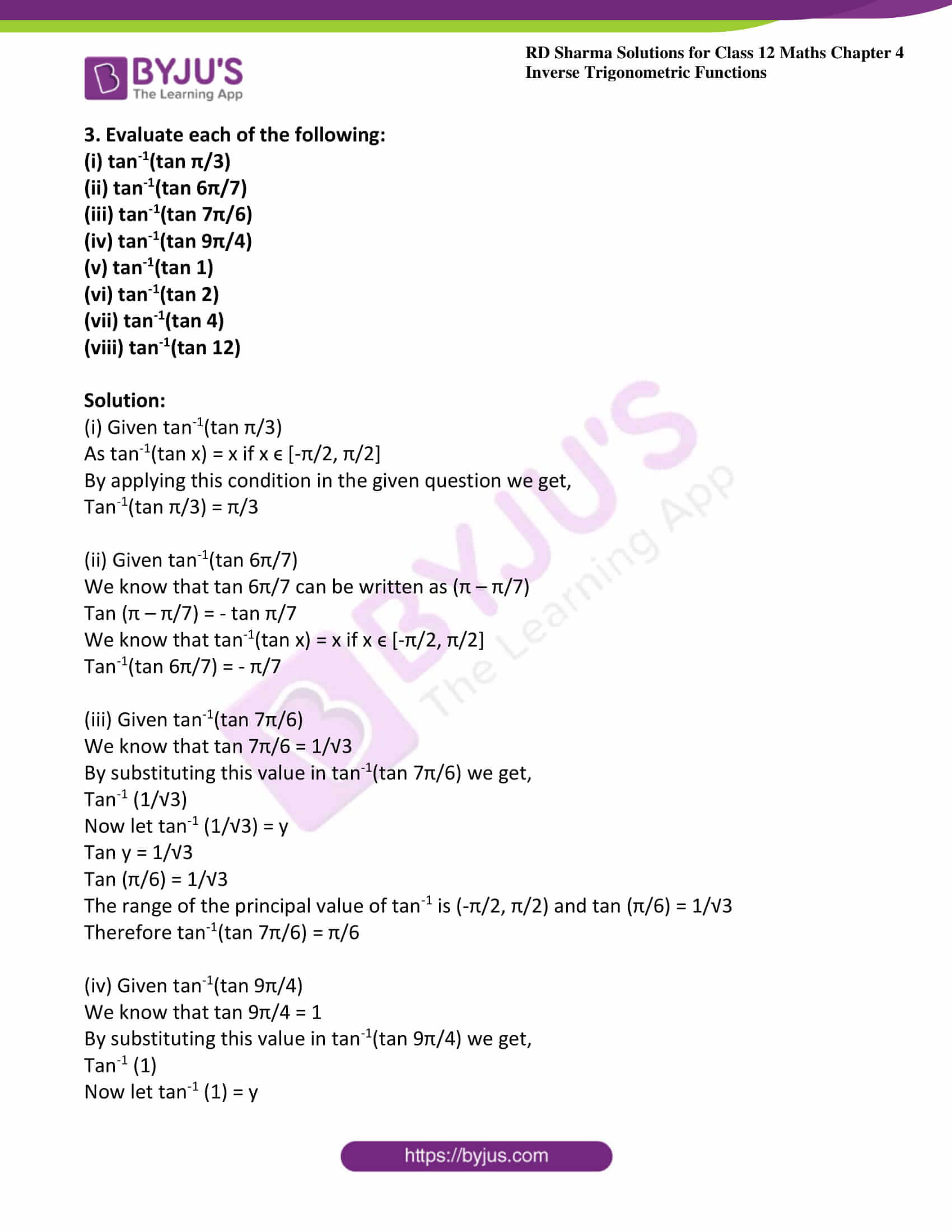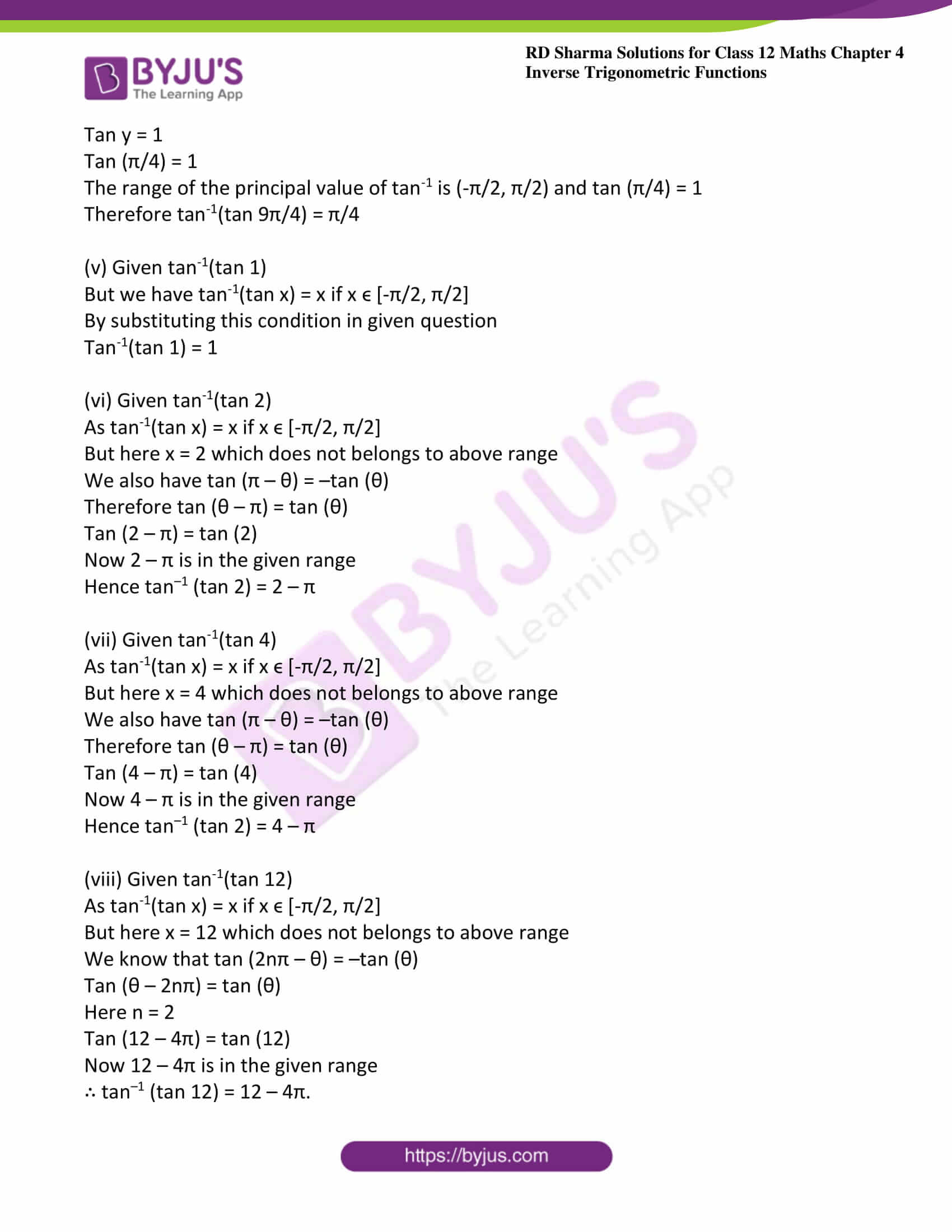### Exercise 4.7 Page No: 4.42

1. Evaluate each of the following:

(i) sin-1(sin π/6)

(ii) sin-1(sin 7π/6)

(iii) sin-1(sin 5π/6)

(iv) sin-1(sin 13π/7)

(v) sin-1(sin 17π/8)

(vi) sin-1{(sin – 17π/8)}

(vii) sin-1(sin 3)

(viii) sin-1(sin 4)

(ix) sin-1(sin 12)

(x) sin-1(sin 2)

Solution:

(i) Given sin-1(sin π/6)

We know that the value of sin π/6 is ½

By substituting this value in sin-1(sin π/6)

We get, sin-1 (1/2)

Now let y = sin-1 (1/2)

Sin (π/6) = ½

The range of principal value of sin-1(-π/2, π/2) and sin (π/6) = ½

Therefore sin-1(sin π/6) = π/6

(ii) Given sin-1(sin 7π/6)

But we know that sin 7π/6 = – ½

By substituting this in sin-1(sin 7π/6) we get,

Sin-1 (-1/2)

Now let y = sin-1 (-1/2)

– Sin y = ½

– Sin (π/6) = ½

– Sin (π/6) = sin (- π/6)

The range of principal value of sin-1(-π/2, π/2) and sin (- π/6) = – ½

Therefore sin-1(sin 7π/6) = – π/6

(iii) Given sin-1(sin 5π/6)

We know that the value of sin 5π/6 is ½

By substituting this value in sin-1(sin 5π/6)

We get, sin-1 (1/2)

Now let y = sin-1 (1/2)

Sin (π/6) = ½

The range of principal value of sin-1(-π/2, π/2) and sin (π/6) = ½

Therefore sin-1(sin 5π/6) = π/6

(iv) Given sin-1(sin 13π/7)

Given question can be written as sin (2π – π/7)

Sin (2π – π/7) can be written as sin (-π/7) [since sin (2π – θ) = sin (-θ)]

By substituting these values in sin-1(sin 13π/7) we get sin-1(sin – π/7)

As sin-1(sin x) = x with x ∈ [-π/2, π/2]

Therefore sin-1(sin 13π/7) = – π/7

(v) Given sin-1(sin 17π/8)

Given question can be written as sin (2π + π/8)

Sin (2π + π/8) can be written as sin (π/8)

By substituting these values in sin-1(sin 17π/8) we get sin-1(sin π/8)

As sin-1(sin x) = x with x ∈ [-π/2, π/2]

Therefore sin-1(sin 17π/8) = π/8

(vi) Given sin-1{(sin – 17π/8)}

But we know that – sin θ = sin (-θ)

Therefore (sin -17π/8) = – sin 17π/8

– Sin 17π/8 = – sin (2π + π/8) [since sin (2π – θ) = -sin (θ)]

It can also be written as – sin (π/8)

– Sin (π/8) = sin (-π/8) [since – sin θ = sin (-θ)]

By substituting these values in sin-1{(sin – 17π/8)} we get,

Sin-1(sin – π/8)

As sin-1(sin x) = x with x ∈ [-π/2, π/2]

Therefore sin-1(sin -π/8) = – π/8

(vii) Given sin-1(sin 3)

We know that sin-1(sin x) = x with x ∈ [-π/2, π/2] which is approximately equal to [-1.57, 1.57]

But here x = 3, which does not lie on the above range,

Therefore we know that sin (π – x) = sin (x)

Hence sin (π – 3) = sin (3) also π – 3 ∈ [-π/2, π/2]

Sin-1(sin 3) = π – 3

(viii) Given sin-1(sin 4)

We know that sin-1(sin x) = x with x ∈ [-π/2, π/2] which is approximately equal to [-1.57, 1.57]

But here x = 4, which does not lie on the above range,

Therefore we know that sin (π – x) = sin (x)

Hence sin (π – 4) = sin (4) also π – 4 ∈ [-π/2, π/2]

Sin-1(sin 4) = π – 4

(ix) Given sin-1(sin 12)

We know that sin-1(sin x) = x with x ∈ [-π/2, π/2] which is approximately equal to [-1.57, 1.57]

But here x = 12, which does not lie on the above range,

Therefore we know that sin (2nπ – x) = sin (-x)

Hence sin (2nπ – 12) = sin (-12)

Here n = 2 also 12 – 4π ∈ [-π/2, π/2]

Sin-1(sin 12) = 12 – 4π

(x) Given sin-1(sin 2)

We know that sin-1(sin x) = x with x ∈ [-π/2, π/2] which is approximately equal to [-1.57, 1.57]

But here x = 2, which does not lie on the above range,

Therefore we know that sin (π – x) = sin (x)

Hence sin (π – 2) = sin (2) also π – 2 ∈ [-π/2, π/2]

Sin-1(sin 2) = π – 2

2. Evaluate each of the following:

(i) cos-1{cos (-π/4)}

(ii) cos-1(cos 5π/4)

(iii) cos-1(cos 4π/3)

(iv) cos-1(cos 13π/6)

(v) cos-1(cos 3)

(vi) cos-1(cos 4)

(vii) cos-1(cos 5)

(viii) cos-1(cos 12)

Solution:

(i) Given cos-1{cos (-π/4)}

We know that cos (-π/4) = cos (π/4) [since cos (-θ) = cos θ

Also know that cos (π/4) = 1/√2

By substituting these values in cos-1{cos (-π/4)} we get,

Cos-1(1/√2)

Now let y = cos-1(1/√2)

Therefore cos y = 1/√2

Hence range of principal value of cos-1 is [0, π] and cos (π/4) = 1/√2

Therefore cos-1{cos (-π/4)} = π/4

(ii) Given cos-1(cos 5π/4)

But we know that cos (5π/4) = -1/√2

By substituting these values in cos-1{cos (5π/4)} we get,

Cos-1(-1/√2)

Now let y = cos-1(-1/√2)

Therefore cos y = – 1/√2

– Cos (π/4) = 1/√2

Cos (π – π/4) = – 1/√2

Cos (3 π/4) = – 1/√2

Hence range of principal value of cos-1 is [0, π] and cos (3π/4) = -1/√2

Therefore cos-1{cos (5π/4)} = 3π/4

(iii) Given cos-1(cos 4π/3)

But we know that cos (4π/3) = -1/2

By substituting these values in cos-1{cos (4π/3)} we get,

Cos-1(-1/2)

Now let y = cos-1(-1/2)

Therefore cos y = – 1/2

– Cos (π/3) = 1/2

Cos (π – π/3) = – 1/2

Cos (2π/3) = – 1/2

Hence range of principal value of cos-1 is [0, π] and cos (2π/3) = -1/2

Therefore cos-1{cos (4π/3)} = 2π/3

(iv) Given cos-1(cos 13π/6)

But we know that cos (13π/6) = √3/2

By substituting these values in cos-1{cos (13π/6)} we get,

Cos-1(√3/2)

Now let y = cos-1(√3/2)

Therefore cos y = √3/2

Cos (π/6) = √3/2

Hence range of principal value of cos-1 is [0, π] and cos (π/6) = √3/2

Therefore cos-1{cos (13π/6)} = π/6

(v) Given cos-1(cos 3)

We know that cos-1(cos θ) = θ if 0 ≤ θ ≤ π

Therefore by applying this in given question we get,

Cos-1(cos 3) = 3, 3 ∈ [0, π]

(vi) Given cos-1(cos 4)

We have cos–1(cos x) = x if x ϵ [0, π] ≈ [0, 3.14]

And here x = 4 which does not lie in the above range.

We know that cos (2π – x) = cos(x)

Thus, cos (2π – 4) = cos (4) so 2π–4 belongs in [0, π]

Hence cos–1(cos 4) = 2π – 4

(vii) Given cos-1(cos 5)

We have cos–1(cos x) = x if x ϵ [0, π] ≈ [0, 3.14]

And here x = 5 which does not lie in the above range.

We know that cos (2π – x) = cos(x)

Thus, cos (2π – 5) = cos (5) so 2π–5 belongs in [0, π]

Hence cos–1(cos 5) = 2π – 5

(viii) Given cos-1(cos 12)

Cos–1(cos x) = x if x ϵ [0, π] ≈ [0, 3.14]

And here x = 12 which does not lie in the above range.

We know cos (2nπ – x) = cos (x)

Cos (2nπ – 12) = cos (12)

Here n = 2.

Also 4π – 12 belongs in [0, π]

∴ cos–1(cos 12) = 4π – 12

3. Evaluate each of the following:

(i) tan-1(tan π/3)

(ii) tan-1(tan 6π/7)

(iii) tan-1(tan 7π/6)

(iv) tan-1(tan 9π/4)

(v) tan-1(tan 1)

(vi) tan-1(tan 2)

(vii) tan-1(tan 4)

(viii) tan-1(tan 12)

Solution:

(i) Given tan-1(tan π/3)

As tan-1(tan x) = x if x ϵ [-π/2, π/2]

By applying this condition in the given question we get,

Tan-1(tan π/3) = π/3

(ii) Given tan-1(tan 6π/7)

We know that tan 6π/7 can be written as (π – π/7)

Tan (π – π/7) = – tan π/7

We know that tan-1(tan x) = x if x ϵ [-π/2, π/2]

Tan-1(tan 6π/7) = – π/7

(iii) Given tan-1(tan 7π/6)

We know that tan 7π/6 = 1/√3

By substituting this value in tan-1(tan 7π/6) we get,

Tan-1 (1/√3)

Now let tan-1 (1/√3) = y

Tan y = 1/√3

Tan (π/6) = 1/√3

The range of the principal value of tan-1 is (-π/2, π/2) and tan (π/6) = 1/√3

Therefore tan-1(tan 7π/6) = π/6

(iv) Given tan-1(tan 9π/4)

We know that tan 9π/4 = 1

By substituting this value in tan-1(tan 9π/4) we get,

Tan-1 (1)

Now let tan-1 (1) = y

Tan y = 1

Tan (π/4) = 1

The range of the principal value of tan-1 is (-π/2, π/2) and tan (π/4) = 1

Therefore tan-1(tan 9π/4) = π/4

(v) Given tan-1(tan 1)

But we have tan-1(tan x) = x if x ϵ [-π/2, π/2]

By substituting this condition in given question

Tan-1(tan 1) = 1

(vi) Given tan-1(tan 2)

As tan-1(tan x) = x if x ϵ [-π/2, π/2]

But here x = 2 which does not belongs to above range

We also have tan (π – θ) = –tan (θ)

Therefore tan (θ – π) = tan (θ)

Tan (2 – π) = tan (2)

Now 2 – π is in the given range

Hence tan–1 (tan 2) = 2 – π

(vii) Given tan-1(tan 4)

As tan-1(tan x) = x if x ϵ [-π/2, π/2]

But here x = 4 which does not belongs to above range

We also have tan (π – θ) = –tan (θ)

Therefore tan (θ – π) = tan (θ)

Tan (4 – π) = tan (4)

Now 4 – π is in the given range

Hence tan–1 (tan 2) = 4 – π

(viii) Given tan-1(tan 12)

As tan-1(tan x) = x if x ϵ [-π/2, π/2]

But here x = 12 which does not belongs to above range

We know that tan (2nπ – θ) = –tan (θ)

Tan (θ – 2nπ) = tan (θ)

Here n = 2

Tan (12 – 4π) = tan (12)

Now 12 – 4π is in the given range

∴ tan–1 (tan 12) = 12 – 4π.

### Access other exercises of RD Sharma Solutions For Class 12 Chapter 4 – Inverse Trigonometric Functions

Exercise 4.1 Solutions

Exercise 4.2 Solutions

Exercise 4.3 Solutions

Exercise 4.4 Solutions

Exercise 4.5 Solutions

Exercise 4.6 Solutions

Exercise 4.8 Solutions

Exercise 4.9 Solutions

Exercise 4.10 Solutions

Exercise 4.11 Solutions

Exercise 4.12 Solutions

Exercise 4.13 Solutions

Exercise 4.14 Solutions